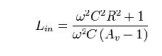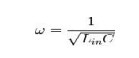Home | | Linear Integrated Circuits | Sine Wave Generators (Oscillators)

# Sine Wave Generators (Oscillators)

Sine wave oscillator circuits use phase shifting techniques that usually employ ┬Ę Two RC tuning networks, and ┬Ę Complex amplitude limiting circuitry

Sine Wave Generators (Oscillators)

Sine wave oscillator circuits use phase shifting techniques that usually employ

┬Ę           Two RC tuning networks, and

┬Ę           Complex amplitude limiting circuitry

## RC Phase Shift OscillatorRC phase shift oscillator using op-amp in inverting amplifier introduces the phase shift of 180┬║ between input and output. The feedback network consists of 3 RC sections each producing 60┬║ phase shift. Such a RC phase shift oscillator using op-amp is shown in the figure.

The output of amplifier is given to feedback network. The output of feedback network drives the amplifier. The total phase shift around a loop is 1800 of amplifier and 180due to 3 RC sections, thus 360 ┬║. This satisfies the required condition for positive feedback and circuit works as an oscillator.The loop phase shift is ŌĆō180┬░ when the phase shift of each section is ŌĆō60┬░, and this occurs when Žē = 2ŽĆf = 1.732/RC because the tangent 60┬░ = 1.73. The magnitude of ╬▓ at this point is (1/2)3, so the gain, A, must be equal to 8 for the system gain to be equal to 1.

## Wien Bridge Oscillator:

Figure 5. 3 give the Wien-bridge circuit configuration. The loop is broken at the positive input, and the return signal is calculated in Equation 2 below.When Žē = 2ŽĆf = 1/RC, the feedback is in phase (this is positive feedback), and the gain is 1/3, so oscillation requires an amplifier with a gain of 3. When RF = 2RG, the amplifier gain is 3 and oscillation occurs at f = 1/2ŽĆRC. The circuit oscillated at 1.65 kHz rather than 1.59 kHz with the component values shown in Figure 3, but the distortion is noticeable.Figure 4 shows a Wien-bridge circuit with non-linear feedback. The lamp resistance, RL, is nominally selected as half the feedback resistance, RF, at the lamp current established by RF and RL. The non-linear relationship between the lamp current and resistance keeps output voltage changes small.

If a voltage source is applied directly to the input of an ideal amplifier with feedback, the input current will be:Where vin is the input voltage, vout is the output voltage, and Zf is the feedback impedance. If the voltage gain of the amplifier is defined as:If Av is greater than 1, the input admittance is a negative resistance in parallel with an inductance.

The inductance is:If a capacitor with the same value of C is placed in parallel with the input, the circuit has a natural resonance at:Substituting and solving for inductance yields:If Av is chosen to be 3: Lin = R2C

Substituting this value yields:Similarly, the input resistance at the frequency above is:For Av = 3:  Rin = ŌłÆ R

If a resistor is placed in parallel with the amplifier input, it will cancel some of the negative resistance. If the net resistance is negative, amplitude will grow until clipping occurs.

Similarly, if the net resistance is positive, oscillation amplitude will decay. If a resistance is added in parallel with exactly the value of R, the net resistance will be infinite and the circuit can sustain stable oscillation at any amplitude allowed by the amplifier.

Increasing the gain makes the net resistance more negative, which increases amplitude. If gain is reduced to exactly 3 when suitable amplitude is reached, stable, low distortion oscillations will result.

Amplitude stabilization circuits typically increase gain until suitable output amplitude is reached. As long as R, C, and the amplifier are linear, distortion will be minimal.

Tags : Waveform Generators , Linear Integrated Circuits : Waveform Generators and Special Function ICs
Study Material, Lecturing Notes, Assignment, Reference, Wiki description explanation, brief detail
Linear Integrated Circuits : Waveform Generators and Special Function ICs : Sine Wave Generators (Oscillators) | Waveform Generators